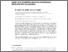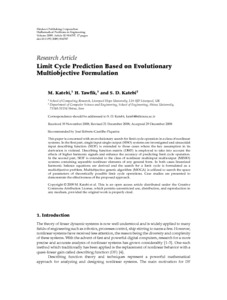# Limit Cycle Prediction Based on Evolutionary Multiobjective Formulation

Katebi, K and Tawfik, Hissam and Katebi, S.D. (2009) Limit Cycle Prediction Based on Evolutionary Multiobjective Formulation. Mathematical Problems in Engineering, 2009. pp. 1-17. ISSN 1563-5147Preview
Text
Paper1.Mathematical.Problems.Engineering.pdf

## Abstract

This paper is concerned with an evolutionary search for limit cycle operation in a class of nonlinear systems. In the first part, single input single output (SISO) systems are investigated and sinusoidal
input describing function (SIDF) is extended to those cases where the key assumption in its
derivation is violated. Describing function matrix (DMF) is employed to take into account the
effects of higher harmonic signals and enhance the accuracy of predicting limit cycle operation.
In the second part, SIDF is extended to the class of nonlinear multiinput multioutput (MIMO)
systems containing separable nonlinear elements of any general form. In both cases linearized
harmonic balance equations are derived and the search for a limit cycle is formulated as a
multiobjective problem. Multiobjective genetic algorithm (MOGA) is utilized to search the space
of parameters of theoretically possible limit cycle operations. Case studies are presented to
demonstrate the effectiveness of the proposed approach.

Item Type: Article Q Science > QA Mathematics > QA75 Electronic computers. Computer science Faculty of Science > Mathematics and Computer Science Users 3 not found. 05 Mar 2014 15:34 09 Sep 2016 13:13 https://hira.hope.ac.uk/id/eprint/249View Item